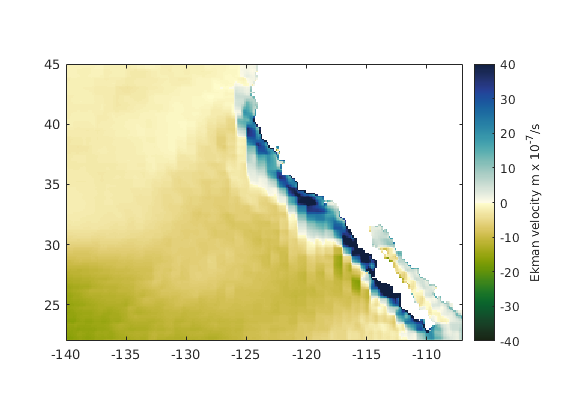# ekman documentation

The ekman function estimates the classical Ekman transport and upwelling/downwelling from 10 m winds.

Back to Climate Data Tools Contents

## Syntax

```[UE,VE,wE] = ekman(lat,lon,u10,v10)
[UE,VE,wE] = ekman(...,'Cd',Cd)
[UE,VE,wE] = ekman(...,'rho',waterDensity)
[UE,VE,wE,dE] = ekman(...)```

## Description

[UE,VE,wE] = ekman(lat,lon,u10,v10) estimates the zonal (UE, m^2/s) and meridional (VE, m^2/s) Ekman layer transports along with vertical velocities (wE, m/s) associated with Ekman pumping. Positive values of wE indicate upwelling. Inputs lat and lon must be 2D grids whose dimensions zonal (u10, m/s) and meridional (v10, m/s) wind speeds taken 10 m above the surface. Wind speeds are automatically converted to wind stress via windstress before performing the Ekman transport calculations. Inputs u10 and v10 can be 2D grids the same size as lat and lon, or they can be 3D matrices whose first two dimensions correspond to lat and lon, with a third dimension corresponding to time.

[UE,VE,wE] = ekman(...,'Cd',Cd) specifies a drag coefficient for wind stress calculation. Cd can be a scalar or a matrix whose dimensions match u10 and v10. Default Cd is 1.25e-3.

[UE,VE,wE] = ekman(...,'rho',waterDensity) specifies water density. Default is 1025 kg/m3.

[UE,VE,wE,dE] = ekman(...) also gives an approximate Ekman layer depth dE.

## Example

Here's some wind and sea surface temperature data. Load and plot the sea surface temperatures and wind. Below I'm using imagescn to make NaN values transparent, but you can use plain old imagesc if you prefer. The colormap is set by the cmocean function (Thyng et al., 2016). To get the RGB values of dark gray I'm using rgb.

```load pacific_wind.mat

figure
imagescn(lon,lat,sst)
axis image      % (trims away extra whitespace & sets aspect ratio to 1:1)
cmocean thermal % sets the colormap
cb = colorbar;
ylabel(cb,' sea surface temperature ({\circ}C) ')

hold on
quiversc(lon,lat,u10,v10,'color',rgb('dark gray'))
```To prevent any possible mixups of dimensions, the ekman function does not accept lats and lons as vectors, so we have to convert lat and lon to 2D grids with meshgrid before calculating Ekman transport.

```[Lon,Lat] = meshgrid(lon,lat);
[UE,VE,wE] = ekman(Lat,Lon,u10,v10);
```
```Warning: You have included some data points within 10 degrees of the equator.
Some folks say Ekman is invalid within 10 degrees of the equator, others say
Ekman's formulas work as close as two or three degrees from the equator. There
is no clearly defined cutoff latitude, but the issue is that Ekman divides by
the Coriolis frequency, which approaches zero at the equator.
```

(Note the warning that appears when latitudes are within 10 degrees of the equator.)

Plot vertical velocities wE after masking-out land values. Conveniently, our sst dataset is already NaN wherever there's land, so we can use sst to make a mask. (If the dataset didn't contain a clear way to distinguish between land and ocean, we could just use island instead.)

```% Mask-out land values:
wE(isnan(sst)) = nan;

figure
imagescn(lon,lat,wE*1e7);
axis image
caxis([-1 1]*40)
cmocean -delta
cb = colorbar;
ylabel(cb,'Ekman velocity m x 10^{-7}/s')
```## Coastal upwelling off California

Upwelling near the California coast is an important process for climate and biology. Let's take a closer look:

```axis([-140 -107 22 45])
```The blue areas correspond to upwelling and are the result of divergent transport of surface water. Let's plot the wind vectors in dark gray as before, but now we'll also plot the Ekman transport as red vectors:

```hold on

% Plot wind vectors:
quiversc(lon,lat,u10,v10,'color',rgb('dark gray'));

% Plot Ekman transport vectors:
quiversc(lon,lat,UE,VE,'color',rgb('bright red'));
```Just as we expect, the surface layer transport is 90 degrees to the right of wind direction in the northern hemisphere, and that's what drives Ekman upwelling.

## Mimicking Kessler

Similarly, let's take a look at the region analyzed by Kessler 2002. We'll recreate Kessler's Figure 6b, which plots upwelling in units of meters per month. There are 2629800 seconds in a month, so we have to multiply wE by that number before contouring. Also, Kessler's color axis is nonlinear, so we'll use their contour values, but I'm not going to worry about stetching and compressing the colorbar to match Kessler.

```cvals = [-40 -20 -10 -5 -2.5 -1 - 2.5 5 10 20 40];

figure
contourf(lon,lat,wE*2629800,cvals);
axis xy image
caxis([-1 1]*40)
cb = colorbar;
ylabel(cb,'Ekman velocity m/month')
axis([-110 -80 8 23])

% Set the colormap:
colormap(rgb('yellow','orange','red','pink','light pink','white',...
'pale blue','light blue','blue','cyan','light cyan'));

borders('countries','facecolor',rgb('gray'))
```Note: Because upwelling and downwelling are two sides of the same coin, it would be best to use a truly divergent, perceptually uniform colormap, such as those provided by the cmocean function. Nonetheless, we're trying to mimic the published plot, so defining the colors with rgb is the easiest solution.

## References

Kessler, William S. "Mean three-dimensional circulation in the northeast tropical Pacific." Journal of Physical Oceanography 32.9 (2002): 2457-2471. doi:10.1175/1520-0485-32.9.2457.

Thyng, K.M., C.A. Greene, R.D. Hetland, H.M. Zimmerle, and S.F. DiMarco. 2016. True colors of oceanography: Guidelines for effective and accurate colormap selection. Oceanography 29(3):9-13, doi:10.5670/oceanog.2016.66.

## Author Info

The ekman function and supporting documentation were written by Chad A. Greene of the University of Texas at Austin (UTIG), February 2017.